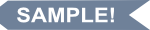# Longitudinal Structural Equation Modeling with Mplus

## A Latent State-Trait Perspective

HardcoverPaperbacke-bookprint + e-book
Hardcover
October 8, 2020
ISBN 9781462544240
Price: \$122.00
344 Pages
Size: 6⅛" x 9¼"
Paperback
October 8, 2020
ISBN 9781462538782
Price: \$62.00
344 Pages
Size: 6⅛" x 9¼"
e-book
October 7, 2020
ePub and PDF ?
Price: \$62.00
344 Pages
print + e-book
Paperback + e-Book (ePub and PDF) ?
Price: \$124.00 \$68.20
344 Pages
See related items for this product

Read the Series Editor's Note by Todd D. Little
List of Abbreviations

Guide to Statistical Symbols

1. A Measurement Theoretical Framework for Longitudinal Data: Introduction to Latent State–Trait Theory

1.1 Introduction

1.2 Latent State–Trait Theory

1.3 Chapter Summary

2. Single-Factor Longitudinal Models for Single-Indicator Data2.1 Introduction

2.2 The Random Intercept Model

2.3 The Random and Fixed Intercepts Model

2.4 The ?-Congeneric Model

2.5 Chapter Summary

3. Multifactor Longitudinal Models for Single-Indicator Data

3.1 Introduction

3.2 The Simplex Model

3.3 The Latent Change Score Model

3.4 The Trait–State–Error Model

3.5 Latent Growth Curve Models

3.6 Chapter Summary

4. Testing Measurement Equivalence in Longitudinal Studies

4.1 Introduction

4.2 The Latent State (LS) Model

4.3 The Latent State Model with Indicator-Specific Residual Factors (LS-IS Model)

4.4 Chapter Summary

5. Multiple-Indicator Longitudinal Models

5.1 Introduction

5.2 Latent State Change (LSC) Models

5.3 The Latent Autoregressive/Cross-Lagged States (LACS) Model

5.4 Latent State–Trait (LST) Models

5.5 Latent Trait Change (LTC) Models

5.6 Chapter Summary

6. Modeling Intensive Longitudinal Data

6.1 Introduction

6.2 Special features of Intensive Longitudinal Data

6.3 Specifying Longitudinal SEMs for Intensive Longitudinal Data

6.4 Chapter Summary

7. Missing Data Handling

7.1 Introduction

7.2 Missing Data Mechanisms

7.3 Maximum Likelihood Missing Data Handling

7.4 Multiple Imputation (MI)

7.5 Planned Missing Data Designs

7.6 Chapter Summary

8. How to Choose between Models and Report the Results

8.1 Model Selection

8.2 Reporting Results

8.3 Chapter Summary